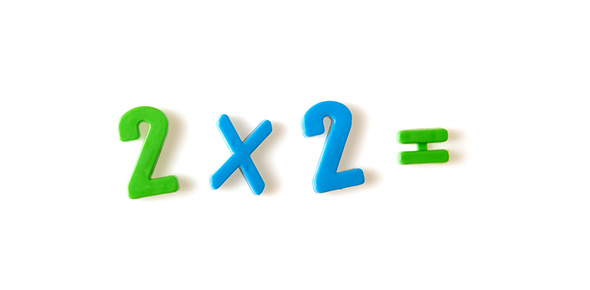# Multiplication Word Problems Quiz

Approved & Edited by ProProfs Editorial Team
At ProProfs Quizzes, our dedicated in-house team of experts takes pride in their work. With a sharp eye for detail, they meticulously review each quiz. This ensures that every quiz, taken by over 100 million users, meets our standards of accuracy, clarity, and engagement.
| Written by NandaAyu
N
NandaAyu
Community Contributor
Quizzes Created: 1 | Total Attempts: 1,105
Questions: 6 | Attempts: 1,106SettingsThis is a complete lesson for third grade with teaching & word problems with the aim of teaching children some basics about multiplication word problems. The basic idea is that we have groups of same size, and children need to just recognize those groups, whether they be towels, pizza slices, balls, or whatever. The word problems in the lesson also involve addition and subtraction so that students need to think, and not apply the operation at hand (multiplication) without even reading the problem.

• 1.

### There are seven rocks in each box. That is a total of  ...

• A.

4 × 7 = 4 + 4 + 4 + 4 + 4 + 4 + 4 = 28 clothes.

• B.

4 × 1 =1 + 1 + 1 + 1 = 4 clothes.

• C.

1 × 4 = 4 clothes.

• D.

4 × 7 = 7 + 7 + 7 + 7 = 28 clothes.

D. 4 × 7 = 7 + 7 + 7 + 7 = 28 clothes.
Explanation
The correct answer is 4 × 7 = 7 + 7 + 7 + 7 = 28 clothes. This is because there are four boxes, and each box contains seven rocks. To find the total number of clothes, we multiply the number of boxes (4) by the number of rocks in each box (7), which gives us 28 clothes.

Rate this question:

• 2.

### Mr. Fidel usually eats four breads a day.  How many breads does he eat in a normal week?Again, we have the situation of EACH DAY the SAME thing happens.7 breads × 4 breads a day = _____ breads in a normal week.

• A.

7 + 7 + 7 + 7 = 28

• B.

14 + 14 = 28

• C.

4 + 4 + 4 + 4 + 4 + 4 + 4 = 28

• D.

28 + 0 = 28

C. 4 + 4 + 4 + 4 + 4 + 4 + 4 = 28
• 3.

### Each puppy's house has three puppies living in it.How many puppies live in the puppy's houses?

• A.

2 × 3 = 3 + 3 = 6 puppies

• B.

3 × 2 = 3 + 3 = 6 puppies

• C.

3 × 2 = 2 + 2 + 2 = 6 puppies

• D.

2 × 3 = 2 + 2 + 2 = 6 puppies

A. 2 × 3 = 3 + 3 = 6 puppies
Explanation
The explanation for the given answer is that each puppy's house has three puppies living in it. So, if we multiply the number of houses (2) by the number of puppies in each house (3), we get a total of 6 puppies. Additionally, we can also add the number of puppies in each house (3 + 3) to get the same result of 6 puppies.

Rate this question:

• 4.

### The SAME amount of something IN EACH thing is often solved by multiplying.

• A.

True

• B.

False

A. True
Explanation
This statement is true because when there is the same amount of something in each thing, it implies that the quantity is consistent and equal. In such cases, multiplying can be used as a mathematical operation to determine the total amount. By multiplying the same amount by the number of things, we can calculate the total quantity. Therefore, the statement is correct.

Rate this question:

• 5.

### Five children are playing volley ball together.They all brought two balls. How many balls do they have total?

• A.

6 × 2 = 6 + 6 = 12 balls

• B.

6 × 2 = 2 + 2 + 2 + 2 + 2 + 2 = 12 balls

• C.

2 × 6 = 6 + 6 = 12 balls

• D.

2 × 6 = 2 + 2 + 2 + 2 + 2 + 2 = 12 balls

B. 6 × 2 = 2 + 2 + 2 + 2 + 2 + 2 = 12 balls
Explanation
The correct answer is obtained by multiplying the number of children (5) by the number of balls each child brought (2). This gives us a total of 10 balls. However, since the question asks for the total number of balls, we need to add the two balls that were already brought by the children, resulting in a total of 12 balls.

Rate this question:

• 6.

### The George family orders four pizzas to eat. Each pizza is sliced into five parts.  How many pizza slices do they get?

• A.

6 × 4 = 4 + 4 + 4 + 4 + 4 + 4 = 24 pizza silces

• B.

6 × 4 = 6 + 6 + 6 + 6 = 24 pizza silces

• C.

4 × 6 = 6 + 6 + 6 + 6 = 24 pizza silces

• D.

4 × 6 = 4 + 4 + 4 + 4 + 4 + 4 = 24 pizza silcesBack to top